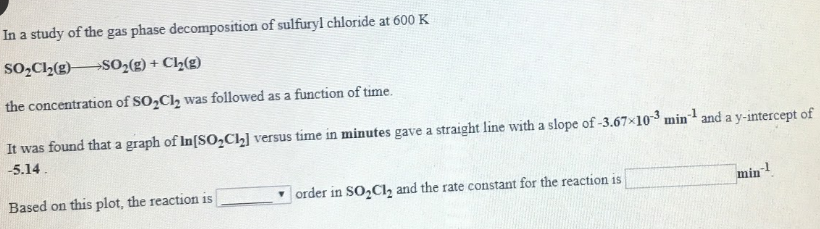# In a study of the gas phase decomposition of sulfuryl chloride at 600 K SO2Cl2(g) → SO2(g) + Cl2(g) the concentration of SOCl2 was followed as a function of time. It was found that a graph of In[SO2Cl2] versus time in minutes gave a straight line with a slope of -3.67x10^-3 min^-1 and a y-intercept of -5.14 Based on this plot, the reaction is ____ order in SO2Cl2 and the rate constant for the reaction is ____ min^-1.